# Ratios

Go back to  'Commercial Math'

 1 Introduction to Ratios 2 What is a Ratio? 3 How to find Ratio? 4 Ratio Formula 5 Tips and Tricks 6 Ratio: Examples and Answers 7 Thinking out of the Box! 8 Practice Questions on Ratios 9 Maths Olympiad Sample Papers 10 Frequently Asked Questions (FAQs) 11 FREE Worksheets on Ratios

We at Cuemath believe that Math is a life skill. Our Math Experts focus on the “Why” behind the “What.” Students can explore from a huge range of interactive worksheets, visuals, simulations, practice tests, and more to understand a concept in depth.

Book a FREE trial class today! and experience Cuemath's LIVE Online Class with your child.

## Introduction to Ratios

We come across several instances in our daily lives where we have to compare two quantities of the same kind.

Grocery shopping is a good example of ratios in daily life.

For example, Mita goes to a grocery store to buy cereal. The shopkeeper gives her two options:

a) $$10\;\text{kg}$$ packet of cereal costs $$\text{Rs.}\; 200$$

b) $$20\;\text{kg}$$ packet of cereal costs $$\text{Rs.}\; 300$$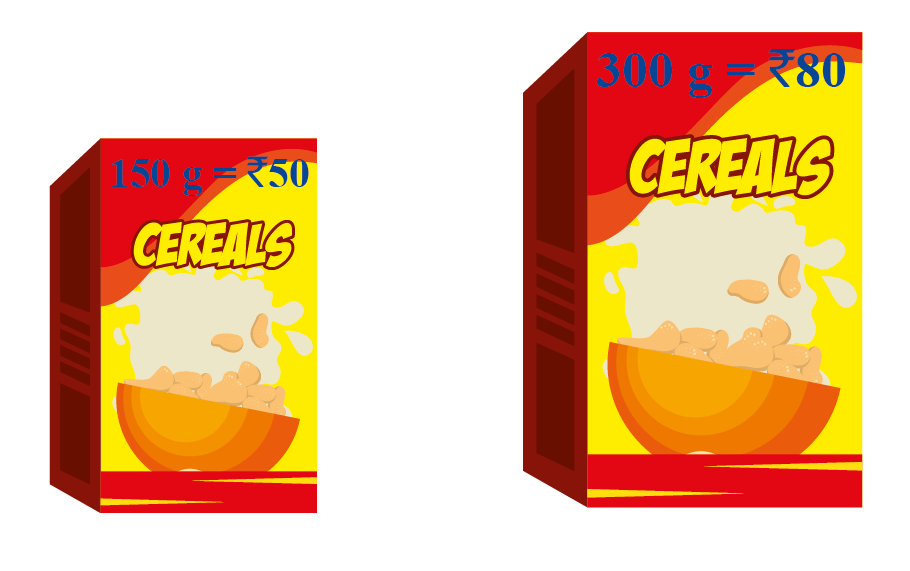Can you help her find the best buy?

By finding the value of each kg of cereal, it will be easy to find the cheaper packet of cereal.

In this instance, comparing by division helps rather than comparing by finding the difference.

## What is a Ratio?

Ratio is a way of comparing two quantities of the same kind by using division.

Let us understand using the following ratio example.

Example 1

There are $$3$$ red squares and $$5$$ blue squares in the following grid.

What is the ratio of red squares to blue squares?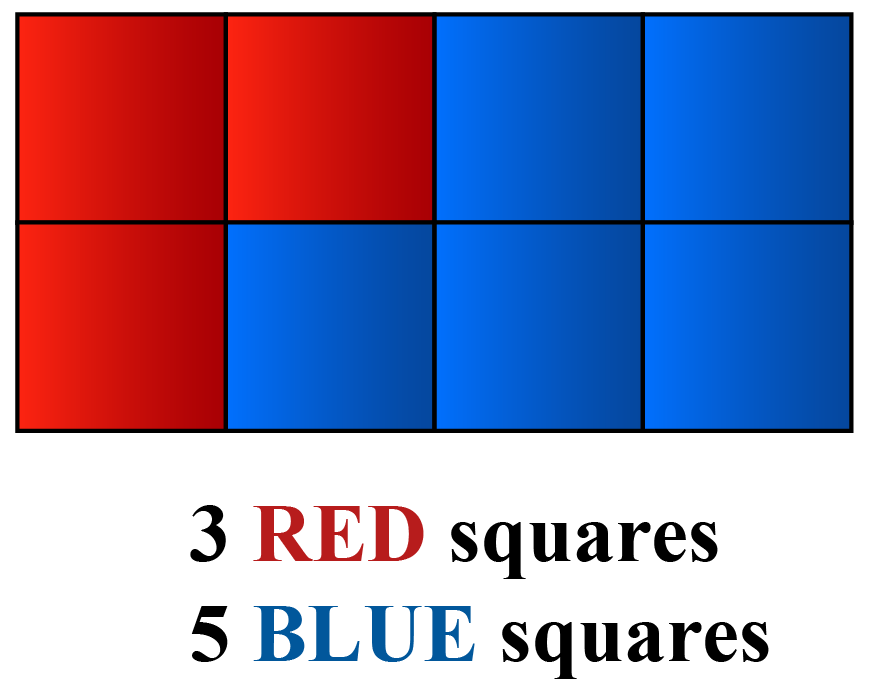Solution:

The ratio of red squares to blue squares can be given as $$3$$ is to $$5$$ OR $$3:5$$ OR \begin{align} \frac{3}{5}\end{align}

Since the question is to find the ratio of red squares to blue squares, the number of red squares is taken as the first term of the ratio.

The number of blue squares is taken as the second term of the ratio.

There are $$3$$ red squares and $$5$$ blue squares.

Thus, the first term of the ratio is $$3$$ and the second term is $$5$$

Now, let’s reverse this.

The ratio of blue squares to red squares can be shown as $$5:3$$

Remember that the ratios $$3:5$$ and $$5:3$$ are not same here! This is because

 \begin{align} \frac{3}{5}\neq \frac{5}{3}\end{align}

### Ratio: Mathematics

Give yourself a try at ratios!

CLUEless in Math? Check out how CUEMATH Teachers will explain Ratios to your kid using interactive simulations & worksheets so they never have to memorise anything in Math again!

Explore Cuemath Live, Interactive & Personalised Online Classes to make your kid a Math Expert. Book a FREE trial class today!

## How to find ratio?

Sofia arranged a get-together at her home and invited four of her friends.

She wanted to serve orange drinks to her friends.

She prepares 5 bottles of orange squash.

The recipe to make orange squash is as follows: "Add 1 cup of orange squash to 3 cups of water to make one bottle of the drink".

How many cups of squash and water will Sofia need to make the drinks?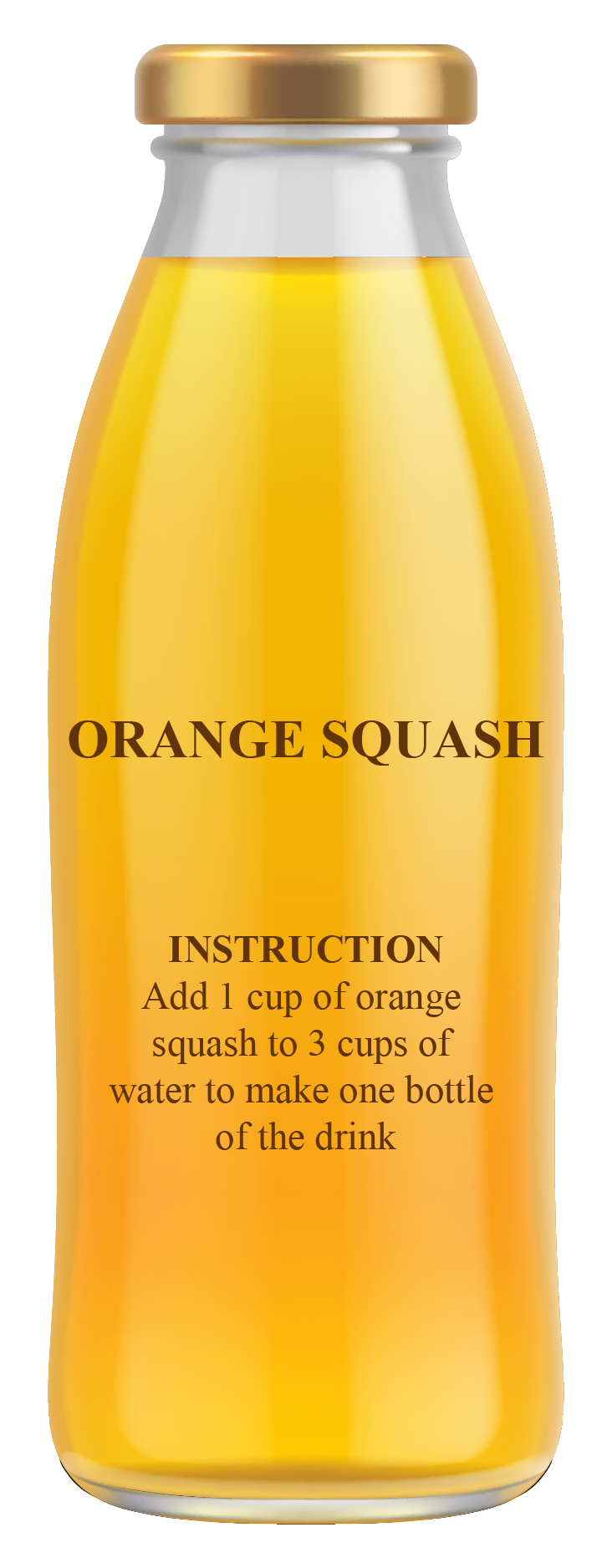Let us find how many cups of squash and water are required to make drinks for Sofia and her friends.

To make the drinks for $$5$$ people, we need $$5$$ times the quantity.

Hence, we multiply the numbers by $$5$$

$1\times 5:3\times 5=5:15$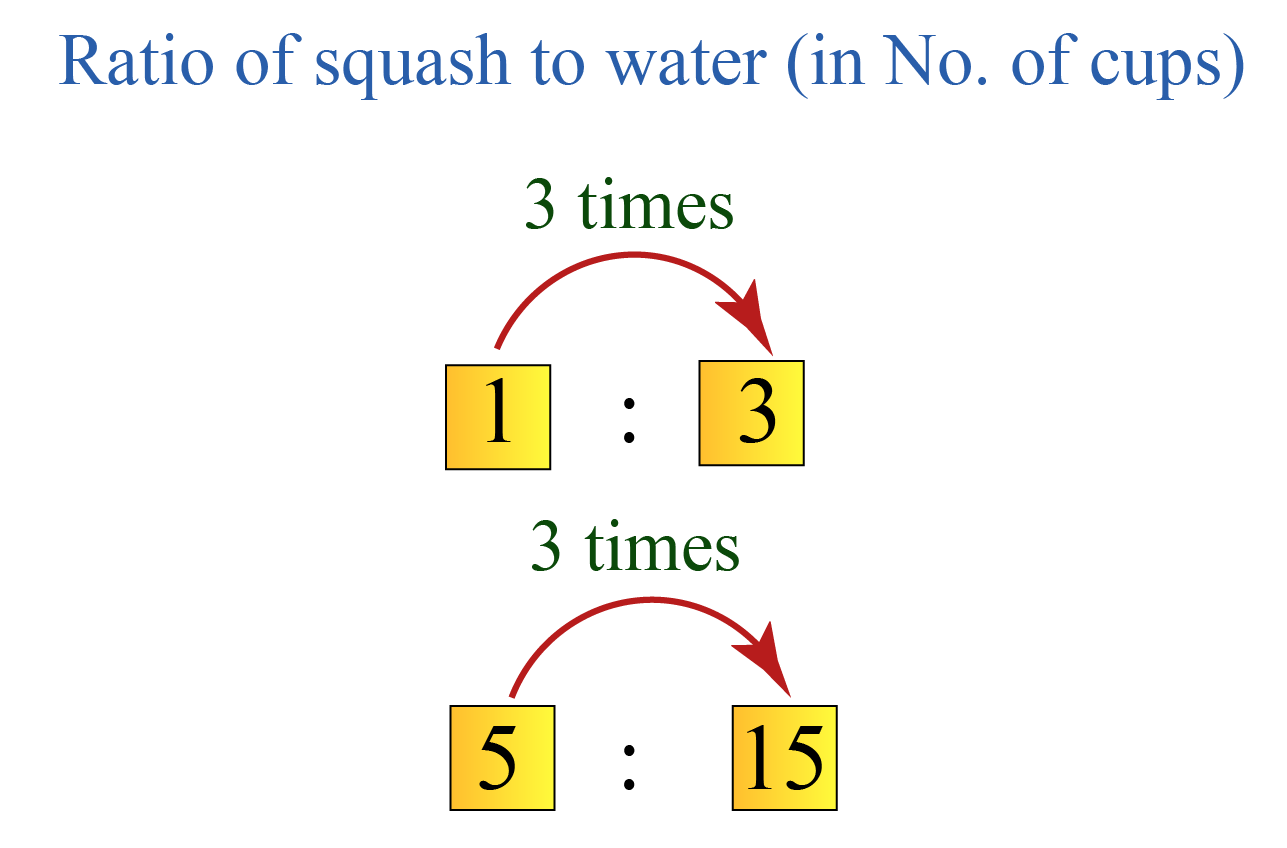Did you observe that the ratio is still the same?

Therefore, the drinks will be just as sweet!

### Equivalent Ratios

The ratios which are obtained by dividing or multiplying by any number are called equivalent ratios.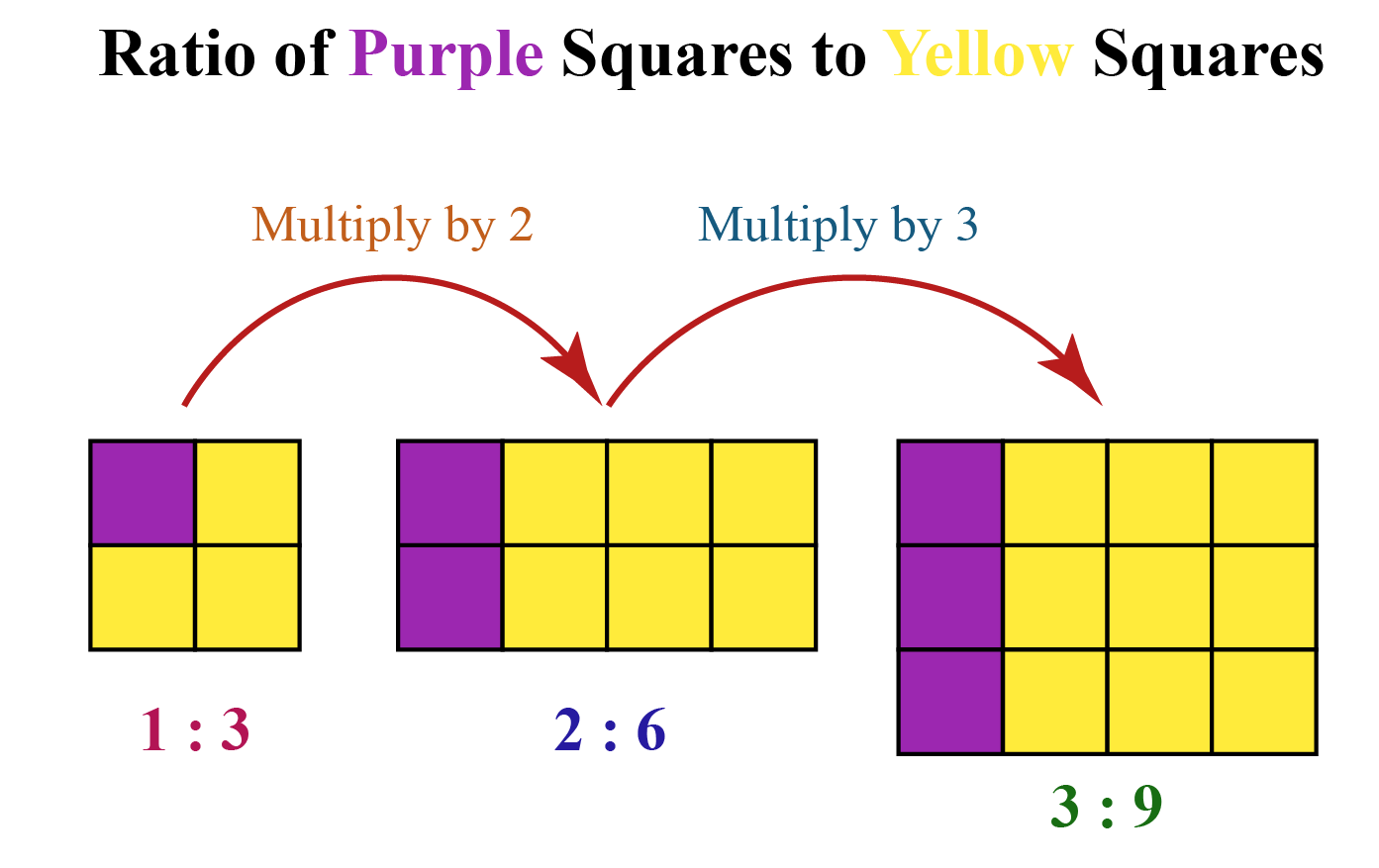Here, the ratios $$1:3$$, $$2:6$$, and $$3:9$$ are equivalent ratios.

The ratio $$1:3$$ is in its lowest form because it can’t be further divided or simplified.

## Ratio: Formula

The ratio formula is used to compare the relationship between two numbers of the same kind.

The ratio formula for two numbers $$a$$ and $$b$$ is given by $$a:b$$ or \begin{align} \frac{a}{b}\end{align}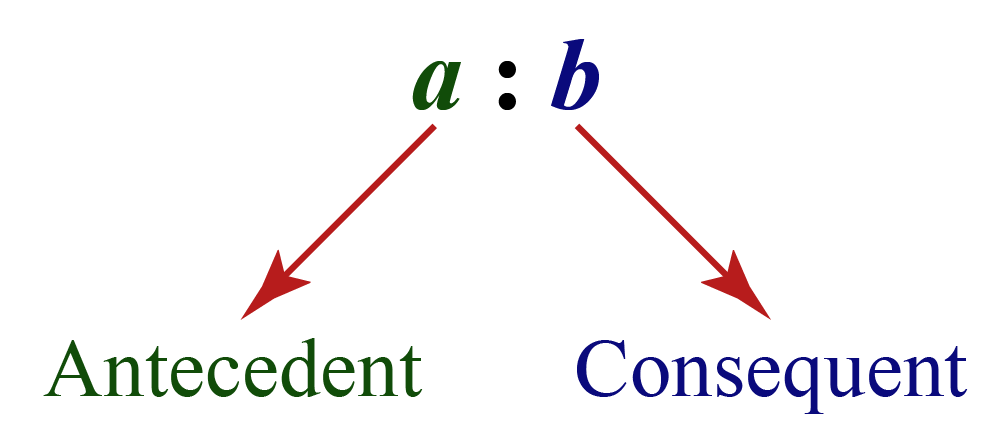Look at the following ratio problem.

Spencer and Oliver decided to start a business together by agreeing to share their profits in the ratio $$2:3$$

By the end of the financial year, they made $$\text{Rs.}\;20,000$$ in profits.Can you tell how much do they each earn in profits and who earns the greater share of the profits?Tips and Tricks
1. If both the numbers $$a$$ and $$b$$ are equal in the ratio $$a:b$$, then $$a:b=1$$

2. If  $$a>b$$ in the ratio $$a:b$$, then $$(a:b)>1$$

3. If  $$a<b$$ in the ratio $$a:b$$, then $$(a:b)<1$$

4. If both the numbers $$a$$ and $$b$$ are multiplied or divided by the same number in the ratio $$a:b$$, then the resulting ratio remains the same as the original ratio.

Help your child score higher with Cuemath’s proprietary FREE Diagnostic Test. Get access to detailed reports, customized learning plans, and a FREE counseling session. Attempt the test now.## Solved Examples

Let us look at a few other examples on ratios to better understand ratio problems.

 Example 1

Gravin has a jar where he keeps $$15$$ marbles out of which $$5$$ are red, $$3$$ are green, $$4$$ are blue and the rest are pink.

Find the ratio of pink marbles to all the marbles.Solution:

There are a total of $$15$$ marbles in the jar out of which $$3$$ are pink marbles.

The required ratio is given by

\begin{align}\frac{\text{Number of pink marbles}}{\text{Total number of marbles}}&=\frac{3}{15}\\&=\frac{1}{5}\end{align}

 Pink marbles $$:$$ All marbles $$=\frac{1}{5}$$
 Example 2

Naira drew a line segment of length $$124\;\text{cm}$$.

She wants to locate the mid-point of this line segment. Can you help her out?

Solution:

Let the point $$P$$ be the mid point of the line segment $$AB$$.

The point $$P$$ divides the line segment in the ratio $$1:1$$

Let us find the length of $$AP$$.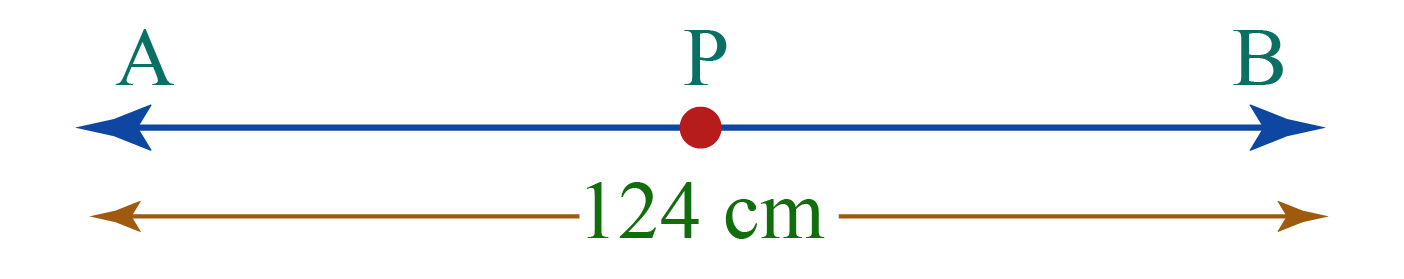Length of AP =\begin{align}\frac{1}{2}\end{align} \times 124=62

 Mid-point is $$62\;\text{cm}$$ from $$A$$.
 Example 3

Jimmy and Jack won a poster-making competition and got a reward of Rs. 2000. What would Jack receive if they share the amount in the ratio 2:3?Solution:

The amount of Rs. 2000 is shared between Jimmy and Jack in the ratio 2:3

So, Jack would get $$\frac{3}{5}$$ portion of the amount Rs. 2000

\begin{align}\text{Amount that Jack received}&=\frac{3}{5}\times2000\\&=3\times400\\&=1200\end{align}

 $$\therefore$$ Jack receives Rs. 1200Think Tank
1. Fill in the following numerator and denominator to make them equivalent ratios.
$\frac{3}{4}=\frac{}{12}=\frac{15}{}$

## Practice Questions

Here are few activities for you to practice. Select/Type your answer and click the "Check Answer" button to see the result.

IMO (International Maths Olympiad) is a competitive exam in Mathematics conducted annually for school students. It encourages children to develop their math solving skills from a competition perspective.

## 1. How do you calculate a ratio?

Ratios are used to make comparisons between two quantities of the same kind.

Ratios can be expressed using the word "to", as a fraction, or with a colon (:).

For example, the ratio of triangles to circles is $$4$$ to $$5$$, \begin{align}\frac{4}{5}\end{align} or $$4:5$$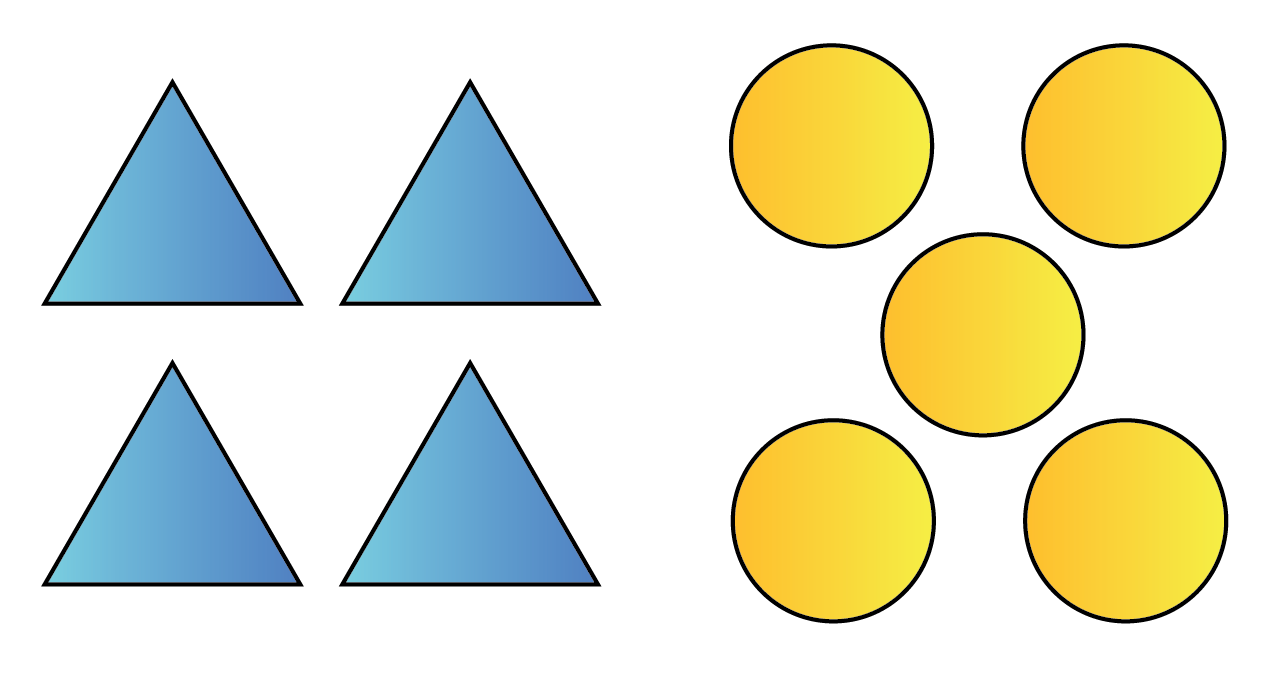## 2. What is the ratio of $$2$$ to $$4$$?

Divide both the terms of the ratio $$2$$ and $$4$$ by the number $$2$$

Thus, the ratio of $$2$$ to $$4$$ is equal to $$1:2$$

## 3.What is basic ratio?

Ratio describes the relationship between two quantities of the same kind.

The ratio between two numbers $$a$$ and $$b$$ is given as $$a:b$$ or \begin{align}\frac{a}{b}\end{align}

Ratio, Proportion, and the Unitary Method